# Miscellaneous Solved Problems in Repetition and Loop - LabVIEW

Rating: 5

8771
1. Create a VI to find the factorial of the given number using For Loop and Shift Registers.
Solution: The front panel has the number and its factorial, while the block diagram contains the codes to solve the problem as shown in Figures P4.1(a) and P4.1(b).

If you would like to Enrich your career with an Android certified professional, then visit Mindmajix - A Global online training platform: “LabVIEW training Course.This course will help you to achieve excellence in this domain.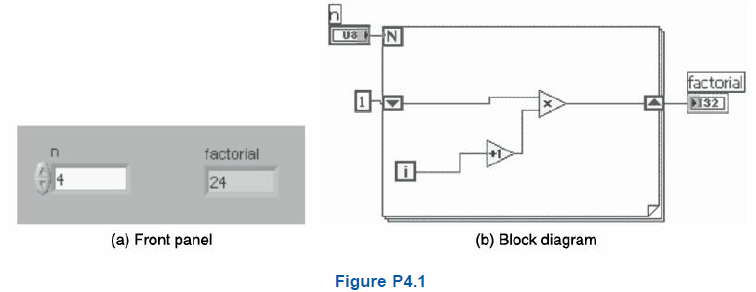2. Create a VI to find the sum of first n natural numbers using a While Loop with a feedback node.
Solution: The front panel and the block diagram to solve the problem are shown in Figures P4.2(a) and P4.2(b). Given a number n, the sum of first n natural numbers is obtained when the program is run.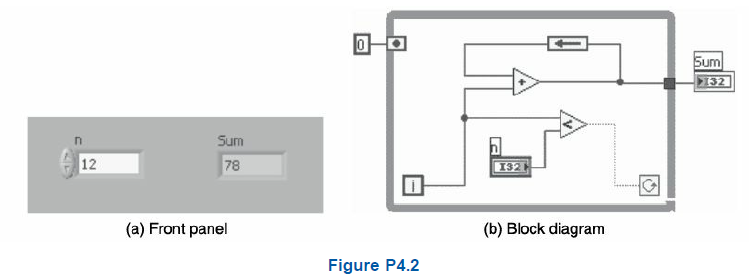3.Create a VI to change the state of the Boolean indicator n times between TRUE and FALSE.
Solution: Build the front panel and the block diagram as shown in Figures P4.3(a) and P4.3(b) to solve the problem.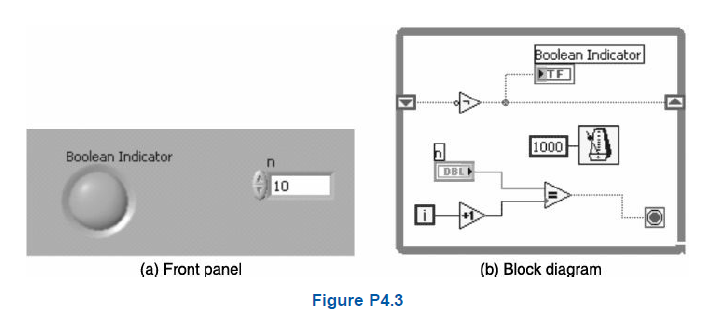4.Create a VI to find the sum of the first 10 natural numbers using a For Loop.
Solution: The front panel and the block diagram to solve the problem are shown in Figures P4.4(a) and P4.4(b).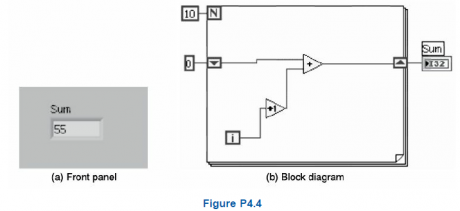5. Create a VI which converts a decimal number to a binary number using For Loops.
Solution: The front panel and the block diagram to convert a decimal number to a binary number are shown in Figures P4.5(a) and P4.5(b).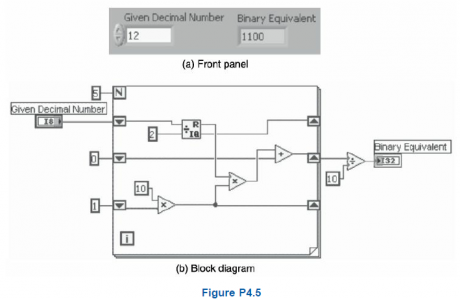6. Create a VI to find the factorial of a given number using a While Loop.
Solution: The front panel and the block diagram to find the factorial are shown in Figures P4.6(a) and P4.6(b).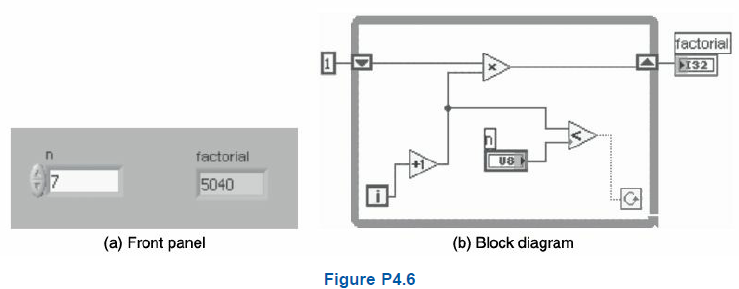7. Create a VI to find ncr and npr of a given number using a For Loop.
Solution: The front panel and the block diagram to find ncr and npr are shown in Figures P4.7(a) and P4.7(b).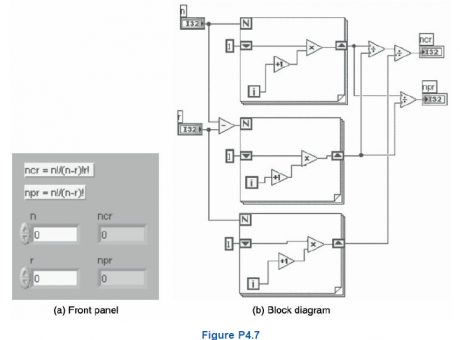8. Create a VI to animate a bird flying. Use the Picture Ring control to insert and display pictures to be animated.

Frequently Asked LabVIEW Interview Questions & Answers

Solution The front panel and the block diagram to solve an animate-a-bird-flying problem are shown in Figures P4.8(a) and P4.8(b).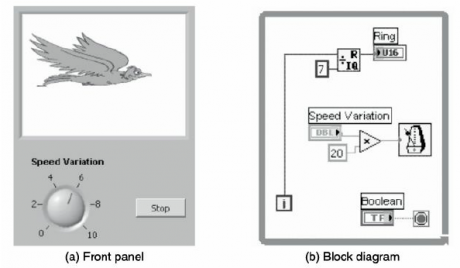9. Create a global VI (Global1.vi) which consists of a knob and a stop button. Create another VI (plot.vi) consisting of a waveform chart. Update the values of Global1.vi’s knob and plot.vi’s slide in the waveform chart. Press the stop button of the Global1.vi to stop both the VIs.
Solution The front panel is shown in Figures P4.9(a) and P4.9(b) and the block diagram to solve the global variable problem are shown in Figure P4.9(c).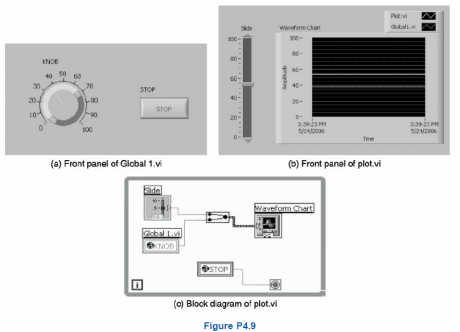10. Using the Global1.vi created in Problem number 9, write the variation of knob values in a file using Write to Measurement File.vi. Stop the VI using the stop button of Global1.vi.
Solution The block diagram to solve the problem is shown in Figure P4.10.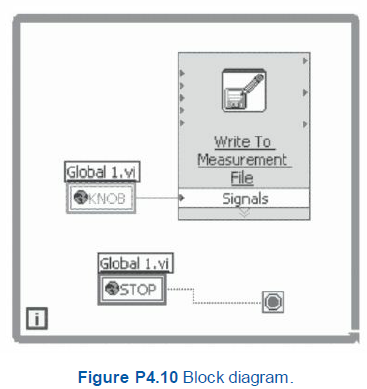11. Create a global VI (Global2.vi) with a waveform chart and a stop button. Update the waveform chart using a random number generator from another VI (chart.vi). Stop the VIs by pressing the stop button of any one of the VIs.
Solutio:n The front panel is shown in Figures P4.11(a) and P4.11(b), and the block diagram to solve the problem is shown in Figure P4.11(c).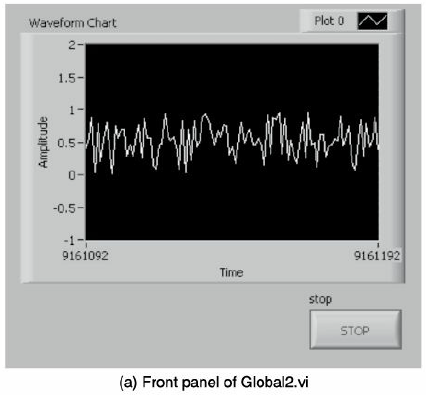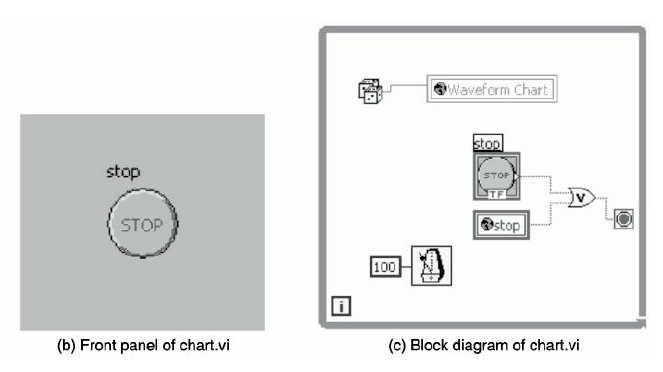Stay updated with our newsletter, packed with Tutorials, Interview Questions, How-to's, Tips & Tricks, Latest Trends & Updates, and more ➤ Straight to your inbox!

Course Schedule
NameDates
LabVIEW TrainingSep 24 to Oct 09
LabVIEW TrainingSep 27 to Oct 12
LabVIEW TrainingOct 01 to Oct 16
LabVIEW TrainingOct 04 to Oct 19
Last updated: 20 September 2022